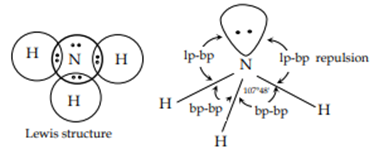# Distorted Geometry↪ The presence of one or more lone pairs of electrons in the valence shell of the central atom gives distorted geometry to the molecules or ion.
Some examples of such molecules are:

Shape of NH3 Molecules:

↪ In NH3, the central atom contains four effective electron pairs but only three of them are bonded pairs.
↪ Since, one of the electron pair is the lone pair, the molecule will have trigonal pyramidal geometry.
↪ Since, lone pair exerts greater repulsion than the bonded pair, the bond angle is less than expected in regular tetrahedral bond angle (109°28'). Actual bond angle is found to be 107.5°.Shape of H2O Molecules:

↪ In H2O, the central atom oxygen has four electron pairs. Hence, expected geometry is tetrahedral but there are two lone pairs and two bond pairs.
↪ Since, there are two lone pairs of electrons around central atom, the actual geometry is bent or angular.
↪ Since lone pair exerts greater repulsion than the bonded pairs, the bond angle is less than the regular tetrahedral bond angle (109°28'). Actual bond angle is found to be 104.5°.Note:
No of e- pair in central atom (O) = 4 Pair
Expected Geometry = Tetrahedral with bond angle 109.28°
No. of lone pair = 2 pairs
No. of bond pair = 2 pairs